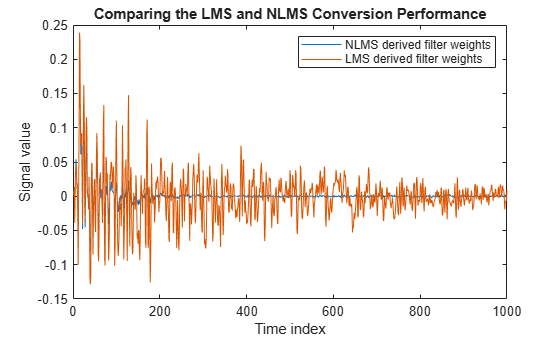## Compare Convergence Performance between LMS Algorithm and Normalized LMS Algorithm

An adaptive filter adapts its filter coefficients inorder to match the coefficients of the unknown system. The objective is to minimize the error signal between the output of the unknown system and the output of the adaptive filter. For the same input, when these two outputs converge and match closely, the coefficients of the two filters are said to match closely. The adaptive filter at this state resembles the unknown system. This example compares the rate at which this convergence happens for the two LMS algorithms - normalized LMS algorithm, LMS algorithm with no normalization.

### Unknown System

Create a `dsp.FIRFilter` that represents the unknown system. Pass the signal, `x`, as an input to the unknown system. The desired signal, `d`, is the sum of the output of the unknown system (FIR filter) and an additive noise signal, `n`.

```filt = dsp.FIRFilter; filt.Numerator = fircband(12,[0 0.4 0.5 1], [1 1 0 0], [1 0.2],... {'w' 'c'}); x = 0.1*randn(1000,1); n = 0.001*randn(1000,1); d = filt(x) + n;```

Choose an adaptation step size of 0.2, and set the length of the adaptive filter to 13 taps.

```mu = 0.2; lms_normalized = dsp.LMSFilter(13,'StepSize',mu,... 'Method','Normalized LMS'); lms_nonnormalized = dsp.LMSFilter(13,'StepSize',mu,... 'Method','LMS');```

Pass the primary input signal, `x`, and the desired signal, `d`, to both the variations of the LMS algorithm. The variables, `e1 `and `e2`, represent the error between the desired signal and the output of the normalized and non normalized filters, respecitvely.

```[~,e1,~] = lms_normalized(x,d); [~,e2,~] = lms_nonnormalized(x,d);```

Plot the error signals for both the variations. When you compare, the error signal for the NLMS variant converges to zero much faster than the error signal for the LMS variant. The normalized version adapts in far fewer iterations to a result almost as good as the non normalized version.

```plot([e1,e2]); title('Comparing the LMS and NLMS Conversion Performance'); legend('NLMS Derived Filter Weights', ... 'LMS Derived Filter Weights','Location', 'NorthEast');```## Related Topics

 Hayes, Monson H., Statistical Digital Signal Processing and Modeling, John Wiley & Sons, 1996, 493–552.

 Haykin, Simon, Adaptive Filter Theory, Prentice-Hall, Inc., 1996

Watch now